Using the XPath Axes

There are 13 axes to master, and we'll take a look at them here, complete with examples. To understand how something like XPath works, there's no better way than seeing it at work as much as possible.

We'll take a look at various examples using XPath Visualiser, and we'll also take a look at some examples using the XPath axes with XSLT. You don't really have to understand the XSLT at this pointyou can just pick out the XPath expression inside the example. But XSLT is important when working with XPath, as we're going to see in Chapter 5, and here it will help us out when XPath Visualiser can't (as with the namespace axis, which XPath Visualiser doesn't display visually). We're already familiar with the child and attribute axes, so we won't introduce them here, but we will introduce all the other axes now, beginning with the ancestor axis.

Using the ancestor Axis

The ancestor axis contains all the ancestors of the context node, including its parents, grandparents, great-grandparents, and so on. This axis always contains the root node (unless the context node is the root node).

Here's an example using XPath Visualiser. In this case, we'll use the location path //planet/day to select the <day> elements in our planetary data example, ch03_01.xml . Then we'll work backward with the ancestor axis to find the <planet> ancestor of each <day> element like this: //planet/day/ancestor::planet . You can see the results in Figure 3.7 (note that we're only searching for <planet> ancestors with this location path, so only <planet> ancestors are selected).

Figure 3.7. Using the ancestor axis.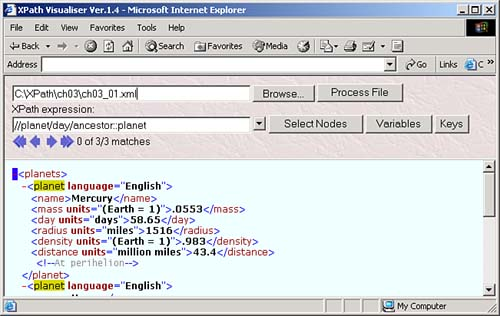Here's an example doing the same thing using XSLT. As discussed in Chapter 1, in XSLT you create a template with an <xsl:template> element to match nodes. In this case, we want to match <day> elements:

<xsl:template match="day">  .   .   .  </xsl:template>

Now we'll use an <xsl:for-each> element to loop over all ancestors of the <day> element, using the XPath ancestor axis:

<xsl:template match="day">  <xsl:for-each select="ancestor::*">   .   .   .   </xsl:for-each>  </xsl:template>

To display the name of the ancestor element, we can use the XSLT <xsl:value-of> element. We can extract the name of the current planet with the XPath expression ./name , where . selects the context node. Here's what that looks like in XSLT:

<?xml version="1.0" encoding="utf-8"?> <xsl:stylesheet version="1.1" xmlns:xsl="http://www.w3.org/1999/XSL/Transform"> <xsl:output method="xml"/> <xsl:template match="day">  <xsl:for-each select="ancestor::*">   <xsl:value-of select="./name"/>   </xsl:for-each>  </xsl:template> <xsl:template match="planet">     <xsl:apply-templates select="day"/> </xsl:template> </xsl:stylesheet>

And here's the result when you use this stylesheet on ch03_01.xml as you can see, we've been able to pick out the names of the ancestors of the <day> elements in our document:

<?xml version="1.0" encoding="utf-8"?>     Mercury     Venus     Earth

Using the ancestor-or-self Axis

The ancestor-or-self axis contains all the ancestors of the context node, and the context node itself. That means, among other things, that this axis always contains the root node.

Here's an example using XPath Visualiser. In this case, we'll use this axis to select all ancestors of <day> elements, as well as the <day> element itself this way: /planet/day/ancestor-or-self::* . You can see the results in Figure 3.8.

Figure 3.8. Using the ancestor-or-self axis.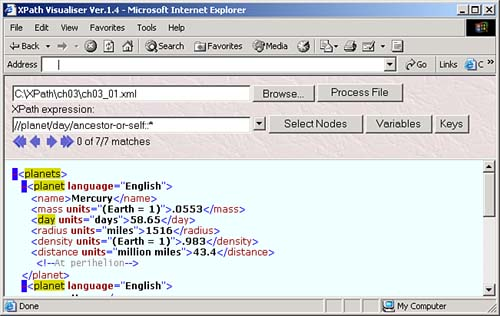Here's an example using XSLT and the ancestor-or-self axis. In this case, we're going to add author attributes set to "Thaddeus" throughout our document like this:

<?xml version="1.0" encoding="utf-8"?> <?xml-stylesheet type="text/xml" href="planets.xsl"?>  <planets author="Thaddeus" >   <planet author="Thaddeus" language="English">  <name>Mercury</name>         <mass units="(Earth = 1)">.0553</mass>  <day author="Thaddeus" units="days">58.65</day>  <radius units="miles">1516</radius>         <density units="(Earth = 1)">.983</density>         <distance units="million miles">43.4</distance><!--At perihelion-->     </planet>  <planet author="Thaddeus" language="English">  <name>Venus</name>         <mass units="(Earth = 1)">.815</mass>         <day units="days">116.75</day>         <radius units="miles">3716</radius>         <density units="(Earth = 1)">.943</density>         <distance units="million miles">66.8</distance><!--At perihelion-->     </planet>     <planet language="English">         <name>Earth</name>         <mass units="(Earth = 1)">1</mass>         <day units="days">1</day>         <radius units="miles">2107</radius>         <density units="(Earth = 1)">1</density>         <distance units="million miles">128.4</distance><!--At perihelion-->     </planet> </planets>

Now say that you want to list by name all ancestors of <day> elements that have an author attributeas well as the current <day> element if it has an author attribute. To do that, you can use the XPath location path ancestor-or-self::*[@author] , which matches all nodes and ancestors that have an author attribute. Here's what it looks like in XSLT:

<?xml version="1.0" encoding="utf-8"?> <xsl:stylesheet version="1.1" xmlns:xsl="http://www.w3.org/1999/XSL/Transform"> <xsl:output method="xml"/> <xsl:template match="day">  <xsl:for-each select="ancestor-or-self::*[@author]">   <xsl:value-of select="local-name(.)"/>   <xsl:text> </xsl:text>   </xsl:for-each>  </xsl:template> <xsl:template match="planet">     <xsl:apply-templates select="day"/> </xsl:template> </xsl:stylesheet>

Here's the result, showing the matching ancestors of all three <day> elements that have author attributes, including the <day> element itself, which has an author attribute:

<?xml version="1.0" encoding="UTF-8"?>  planets planet day   planets planet   planets

Using the descendant Axis

The descendant axis contains all the descendants of the context node. Note that this does not include any attributes or namespace nodes.

Here's an example using XPath Visualiser. In this case, we'll select all descendants of <planet> elements with the location path //planet/descendant::* , as you see in Figure 3.9.

Figure 3.9. Using the descendant axis.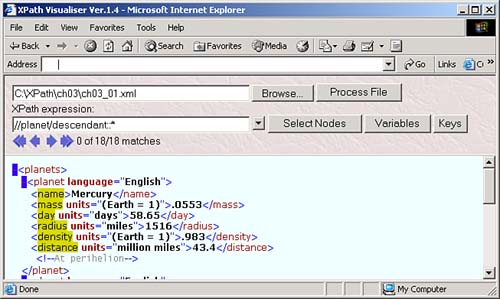Here's an example using XSLT. In this case, we'll check a document to see if it includes a <planet> element for Mercury, and if so, we'll include this element in the result: <info>Sorry, Mercury cannot be found at this time.</ info > . To match Mercury's <planet> element, all you have to do is to check whether any text node descendant of a <planet> element holds the string "Mercury" this way:

<?xml version="1.0" encoding="utf-8"?> <xsl:stylesheet version="1.0" xmlns:xsl="http://www.w3.org/1999/XSL/Transform"> <xsl:output method="xml"/>  <xsl:template match="planet[descendant::text()='Mercury']">   <info>Sorry, Mercury cannot be found at this time.</info>   </xsl:template>  <xsl:template match="*">       <xsl:apply-templates select="*"/>   </xsl:template> </xsl:stylesheet>

That's all it takes. Here's the result, showing the <info> element:

<?xml version="1.0" encoding="utf-8"?>  <info>Sorry, Mercury cannot be found.</info>

Using the descendant-or-self Axis

The descendant-or-self axis contains all the descendants of the context node, and the context node itself. Note, however, that it does not contain any attributes or namespace nodes.

You can see an example in Figure 3.10, where we're selecting all <planet> elements and their descendants with the XPath location path //planet/descendant-or-self::* .

Figure 3.10. Using the descendant-or-self axis.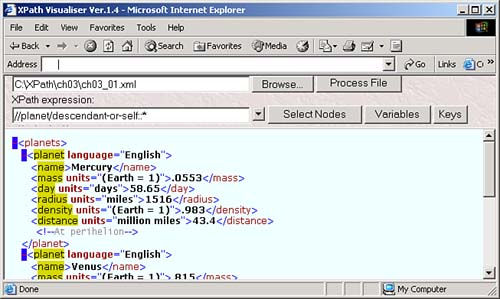Here's an example doing the same thing using XSLT. In this case, we'll use an XSLT template to match all <planet> elements and then loop over all nodes in the node-set returned by using the descendant-or-self axis, displaying each node's name:

<?xml version="1.0" encoding="utf-8"?> <xsl:stylesheet version="1.0" xmlns:xsl="http://www.w3.org/1999/XSL/Transform"> <xsl:output method="xml"/>   <xsl:template match="planet">  <xsl:for-each select="descendant-or-self::*">   <xsl:value-of select="local-name()"/>   <xsl:text> </xsl:text>   </xsl:for-each>  </xsl:template> </xsl:stylesheet>

That's all it takes. Here's the result, where we've been able to list the name of all the descendants of <planet> elements, as well as the <planet> elements themselves , using the descendant-or-self axis:

<?xml version="1.0" encoding="UTF-8"?>     planet name mass day radius density distance     planet name mass day radius density distance     planet name mass day radius density distance

Using the following Axis

The following axis contains all nodes that come after the context node in document order, excluding any of the context node's descendantsand also excluding attribute nodes and namespace nodes.

You can see an example in the XPath Visualiser in Figure 3.11, where we're using this axis to select the following elements after the <mass> element in the first <planet> element, using the XPath location path /planets/planet/mass/following::* .

Figure 3.11. Using the following axis to extract data.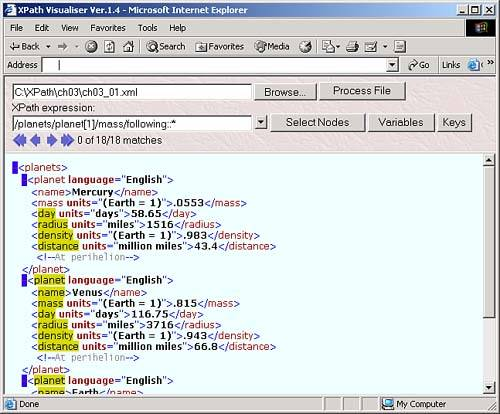Here's an example using XSLT to do the same thing. In this case, we're matching the first <planet> element in an XSLT template and displaying the names of the following elements:

<?xml version="1.0" encoding="utf-8"?> <xsl:stylesheet version="1.1" xmlns:xsl="http://www.w3.org/1999/XSL/Transform"> <xsl:output method="xml"/> <xsl:template match="planet">  <xsl:for-each select="mass/following::*">   <xsl:value-of select="local-name()"/>   <xsl:text> </xsl:text>   </xsl:for-each>  </xsl:template>   <xsl:template match="*">       <xsl:apply-templates select="*"/>   </xsl:template> </xsl:stylesheet>

Here's what the result looks like. Note that we've been able to get all the elements following the <mass> element in the first <planet> element, and then all the following elements in the rest of the document:

<?xml version="1.0" encoding="UTF-8"?> day radius density distance planet name mass day radius density distance planet name mass day radius density distance

Using the following-sibling Axis

The following-sibling axis contains all the following siblings of the context node. You can see an example in the XPath Visualiser in Figure 3.12, where we're using the XPath location path /planets/planet/mass/following-sibling::* to select all following sibling nodes of the <mass> element in the first <planet> element.

Figure 3.12. Using the following-sibling axis.Here's how this example works in XSLT; in this case, we're also matching the first <planet> element's <mass> element and then getting its following sibling elements:

<?xml version="1.0" encoding="utf-8"?> <xsl:stylesheet version="1.1" xmlns:xsl="http://www.w3.org/1999/XSL/Transform"> <xsl:output method="xml"/> <xsl:template match="planet">  <xsl:for-each select="mass/following-sibling::*">   <xsl:value-of select="local-name()"/>   <xsl:text> </xsl:text>   </xsl:for-each>  </xsl:template>   <xsl:template match="*">       <xsl:apply-templates select="*"/>   </xsl:template> </xsl:stylesheet>

Here's the resultas you can see, we've caught all the siblings following the <mass> element in the first <planet> element:

<?xml version="1.0" encoding="UTF-8"?> day radius density distance

Using the namespace Axis

The namespace axis contains the namespace nodes of the context nodenote that the axis will be empty unless the context node is an element. An element will have a namespace node for

• Every attribute of the element whose name starts with "xmlns:".

• Every attribute of an ancestor element whose name starts "xmlns:" (unless, of course, the element itself or a nearer ancestor redeclares the namespace).

• An xmlns attribute, if the element, or some ancestor, has an xmlns attribute.

XPath Visualiser doesn't handle this axis visually, so we'll rely on XSLT here. Here, we'll add an XML namespace declaration to the <planets> element, using the namespace "http://www.XPathCorp.com" like this:

<?xml version="1.0" encoding="utf-8"?> <?xml-stylesheet type="text/xml" href="planets.xsl"?>  <planets xmlns="http://www.XPathCorp.com">  <planet>         <name>Mercury</name>         <mass units="(Earth = 1)">.0553</mass>         <day units="days">58.65</day>         <radius units="miles">1516</radius>         <density units="(Earth = 1)">.983</density>         <distance units="million miles">43.4</distance><!--At perihelion-->     </planet>         .         .         .

In XSLT, we can check the namespaces used in the <planets> element like this:

<?xml version="1.0" encoding="utf-8"?> <xsl:stylesheet version="1.1" xmlns:xsl="http://www.w3.org/1999/XSL/Transform"> <xsl:output method="xml"/>  <xsl:template match="planets">   <xsl:value-of select="namespace::*"/>   </xsl:template>  </xsl:stylesheet>

And here's the result, showing that we can indeed pick out the namespace:

<?xml version="1.0" encoding="UTF-8"?> http://www.XPathCorp.com

Using the parent Axis

The parent axis contains the parent (and only the parent) of the context node, if there is one.

You can see an example in XPath Visualiser in Figure 3.13. Here, we're picking out the parent elements of all <day> elements with the XPath location path //day/parent::* .

Figure 3.13. Using the parent axis to extract data.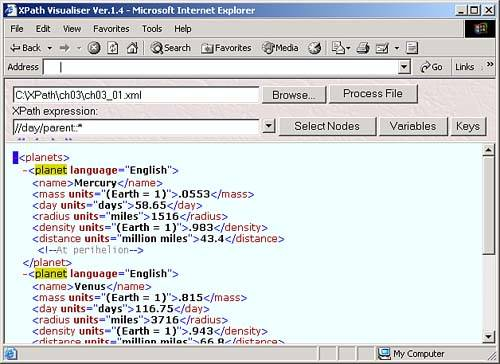And here's the same example in XPath. In this case, we'll match all <day> elements and get the names of their parent elements. Here's what it looks like:

<?xml version="1.0" encoding="utf-8"?> <xsl:stylesheet version="1.1" xmlns:xsl="http://www.w3.org/1999/XSL/Transform"> <xsl:output method="xml"/>  <xsl:template match="//day">   <xsl:for-each select="parent::*">   <xsl:value-of select="local-name()"/>   <xsl:text> </xsl:text>   </xsl:for-each>   </xsl:template>  <xsl:template match="*">     <xsl:apply-templates select="*"/> </xsl:template> </xsl:stylesheet>

And here's the result:

<?xml version="1.0" encoding="UTF-8"?> planet planet planet

USING THE ABBREVIATION ..

Remember that you can also use the abbreviation .. to stand for the parent of the context node.

Using the preceding Axis

The preceding axis contains all nodes that are before the context node in document order, excluding any ancestors of the context node, and also excluding attribute nodes and namespace nodes.

Here's an example using XPath Visualiser. In this case, we'll select all elements preceding the <density> element in the first planet element with the XPath location path //planet/density/preceding::* , as you can see in Figure 3.14.

Figure 3.14. Using the preceding axis to select elements.Let's give this axis a try in XSLT. In this case, say that we want to set the content of the <distance> element to the text "This planet is farther than Mercury from the sun." if the current planet is indeed farther from the sun than Mercury. One way to do that is to see if Mercury comes before the current planet in document order, using the preceding axis:

<?xml version="1.0" encoding="utf-8"?> <xsl:stylesheet version="1.1" xmlns:xsl="http://www.w3.org/1999/XSL/Transform"> <xsl:output method="xml"/>  <xsl:template match="distance[preceding::*/name='Mercury']">   <distance>This planet is farther than Mercury from the sun.</distance>   </xsl:template>  <xsl:template match="@*node()">     <xsl:copy>       <xsl:apply-templates select="@*node()"/>     </xsl:copy>   </xsl:template> </xsl:stylesheet>

If the current planet does come after Mercury, this example inserts the message in its <distance> element, as you see in this result:

<?xml version="1.0" encoding="utf-8"?> <?xml-stylesheet type="text/xml" href="planets.xsl"?> <planets>     <planet>         <name>Mercury</name>         <mass units="(Earth = 1)">.0553</mass>         <day units="days">58.65</day>         <radius units="miles">1516</radius>         <density units="(Earth = 1)">.983</density>         <distance units="million miles">43.4</distance>         <!--At perihelion-->     </planet>     <planet>         <name>Venus</name>         <mass units="(Earth = 1)">.815</mass>         <day units="days">116.75</day>         <radius units="miles">3716</radius>         <density units="(Earth = 1)">.943</density>  <distance>This planet is farther than Mercury from the sun.</distance>  <!--At perihelion-->     </planet>     <planet>         <name>Earth</name>         <mass units="(Earth = 1)">1</mass>         <day units="days">1</day>         <radius units="miles">2107</radius>         <density units="(Earth = 1)">1</density>  <distance>This planet is farther than Mercury from the sun.</distance>  <!--At perihelion-->     </planet> </planets>

Using the preceding-sibling Axis

The preceding-sibling axis contains all the preceding siblings of the context node. Note that if the context node is an attribute node or namespace node, the preceding-sibling axis won't hold anything.

You can see an example in the XPath Visualiser in Figure 3.15, where we're using the XPath location path //planet/preceding-sibling::* to select all preceding siblings of the second <planet> element. Note that just the first <planet> element is selected. On the other hand, if we had used //planet/preceding::* , not only would the first <planet> element be selected, but all that element's child elements would be selected as well.

Figure 3.15. Using the preceding-sibling axis to extract data.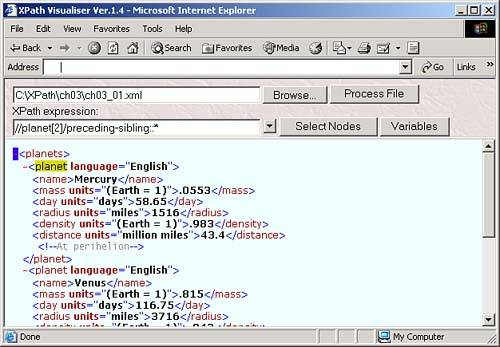Here's a more advanced example using XSLT. In this case, we'll replace the <distance> element in Mercury's <planet> element with <distance>This planet is the closest to the sun.</distance> . If we're matching <distance> elements, how can we make sure that we've got Mercury's <distance> element? We can check the current <distance> element's preceding siblings and look for the text "Mercury". Here's what it looks like in XSLT:

<?xml version="1.0"?> <xsl:stylesheet version="1.0" xmlns:xsl="http://www.w3.org/1999/XSL/Transform"> <xsl:output method="xml"/>  <xsl:template match="distance[preceding-sibling::*='Mercury']">   <distance>This planet is the closest to the sun.</distance>   </xsl:template>  <xsl:template match="@*node()">     <xsl:copy>       <xsl:apply-templates select="@*node()"/>     </xsl:copy>   </xsl:template> </xsl:stylesheet>

And here's the result:

<?xml version="1.0" encoding="utf-8"?> <?xml-stylesheet type="text/xml" href="planets.xsl"?> <planets>     <planet language="English">         <name>Mercury</name>         <mass units="(Earth = 1)">.0553</mass>         <day units="days">58.65</day>         <radius units="miles">1516</radius>         <density units="(Earth = 1)">.983</density>  <distance>This planet is the closest to the sun.</distance>  <!--At perihelion-->     </planet>     <planet language="English">         <name>Venus</name>         <mass units="(Earth = 1)">.815</mass>         <day units="days">116.75</day>         <radius units="miles">3716</radius>         <density units="(Earth = 1)">.943</density>         <distance units="million miles">66.8</distance><!--At perihelion-->     </planet>     <planet language="English">         <name>Earth</name>         <mass units="(Earth = 1)">1</mass>         <day units="days">1</day>         <radius units="miles">2107</radius>         <density units="(Earth = 1)">1</density>         <distance units="million miles">128.4</distance><!--At perihelion-->     </planet> </planets>

Using the self Axis

The self axis contains just the context node, and you can abbreviate "self::node()" as "." . This is a useful axis to know about, because as you know, if you omit the axis, the default is child:: , but sometimes you want to refer to the current node instead. For example, [self::planet] is true only if the context node is a <planet> element.

You can see an example using this axis in XPath Visualiser in Figure 3.16, where we're using the XPath location path //*[self::radius] to select <radius> elements in ch03_01.xml (this location path is equivalent to //radius ).

Figure 3.16. Using the self axis to select <radius> elements.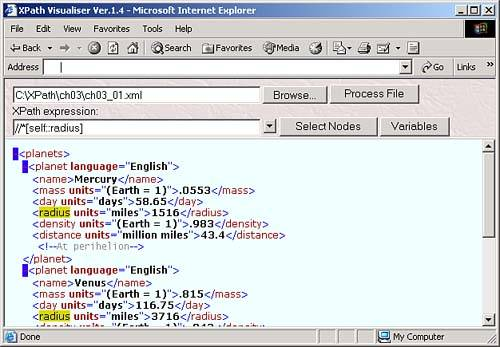Here's an example using XSLT. In this case, we'll use one template to match both <name> and <day> elements in the same template. We can do that by matching name day in a template like this (more on how this works in the next section):

<xsl:template match="name  day">     .     .     . </xsl:template>

At this point, we've matched both <name> and <day> elementsbut suppose that in the body of the template we actually want to treat these elements differently. To do that, we have to check if we're dealing with a <name> element or a <day> element, which we can do with the XSLT element <xsl:if> , where you assign the condition to test to this element's test attribute. Here's what it looks like in XSLT:

<xsl:template match="name  day">  <xsl:if test="self::name">   <xsl:value-of select="."/>   </xsl:if>   <xsl:if test="self::day">   <xsl:value-of select="."/>   <xsl:text> </xsl:text>   <xsl:value-of select="@units"/>   </xsl:if>  </xsl:template>     .     .     .

So now we've taken a look at all 13 axes, from the ancestor axis to the self axis. Note that you can combine location paths with the operatorwe'll take a closer look at that now.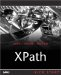XPath Kick Start: Navigating XML with XPath 1.0 and 2.0
ISBN: 0672324113
EAN: 2147483647
Year: 2002
Pages: 131

Similar book on Amazon